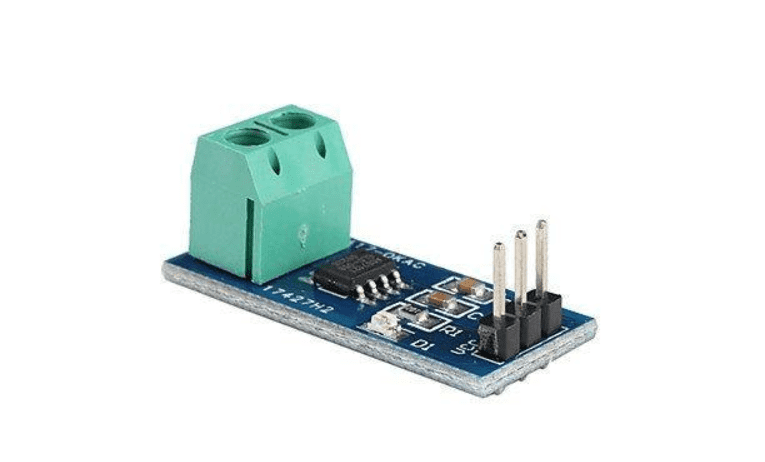# Current/Voltage Sensor

A voltage sensor is used to measure the voltage of an electrical circuit or system.

A current sensor is a device that is used to measure the flow of electrical current in a circuit.## 11 products

#### What is a Current-voltage sensor?

A current sensor is a device that detects electric current in a wire and generates a signal proportional to that current. The generated signal could be analog voltage or current or even a digital output.

#### How do Current-voltage sensors work?

The current voltage sensor works on the principle that detects and converts current to get an output voltage, which is directly proportional to the current in the designed path. This means that when the current passes through the circuit, the voltage drops across the path in which the current is flowing. This creates a magnetic field near the current carrying conductor.

#### What is the use of Current-voltage sensors?

Current voltage sensor devices have major applications in detecting the circuit faults, voltage drops, presence of current and many more. The primary function of voltage sensors is to detect and measure AC and/or DC voltage levels. When the presence of voltage is detected, the sensors provide an output in the form of analogue voltage signals, current levels, frequency and modulated frequency outputs or audible sounds.

Some of them are listed below:

Applications of Current Voltage Sensors

Detection of Power Failure

Power demand control

Detection of Faults in a Circuit

Temperature control

#### What are the types of Current-voltage sensors?

There are mainly two types of voltage sensors:

• Capacitive type voltage sensor: The capacitive voltage sensor works when you hold the barrel in your hand and place the tip near a live conductor, you are inserting the high impedance sensing element into a capacitively coupled series circuit.
• Resistive Voltage Sensors: These voltage sensors have two circuits like a voltage divider & bridge circuit. A voltage supply is applied to this circuit. The output voltage can be decided by the resistance used in the circuit. So the voltage change can be amplified.

Other Sensors